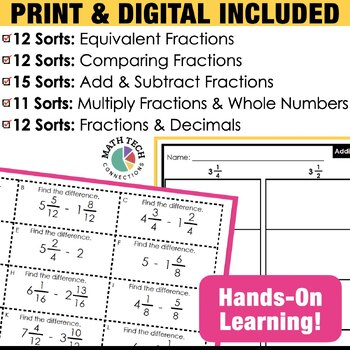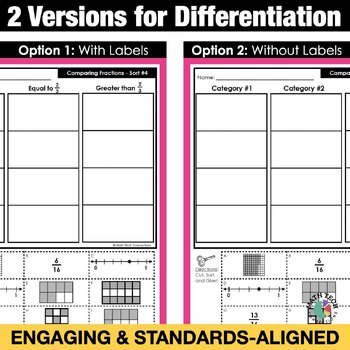# 4th Grade Math Centers Fractions & Decimals Math Sorts Interactive Math Notebook4th, Homeschool
Subjects
Standards
Resource Type
Formats Included
• Zip
Pages
50 + Organization

#### Also included in

1. Math Sorting activities are engaging and encourage critical thinking! Easily differentiate this interactive math activity by using the sorts with or without the labels. Save \$20 when you purchase this bundle.Ways to use: math warm ups, small group lesson, independent math center, partner activity, m
\$25.00
\$45.00
Save \$20.00
2. This third grade math bundle includes 3 best-selling math bundles! Included: Math Tri-Folds, Math Sorts, and Math Morning Work!This is a MUST have resource for your 4th grade classroom. The resources included are flexible and may be used for morning work, whole group practice, guided math practice,
\$60.50
\$121.00
Save \$60.50
3. This 4th grade math bundle includes no-prep math sorting activities that can be used as partner math centers, math warm ups, or math exit tickets. This 4th grade math practice bundle includes both PRINT and DIGITAL format. - The printable format includes 93 cut-and-past activities- The paperless for
\$45.00
\$90.00
Save \$45.00

### Description

These math centers are engaging, interactive activities that encourage math talk. Students explain how they sort their cards and build strong math concepts as they create cards of their own.

* 4th Grade Math Standards Covered *

- 4.NF.1

Sort 1: Benchmark Fractions

Sort 2: Equivalent Fractions

Sort 3: Equivalent Fractions

Sort 4: Equivalent Fractions

- 4.NF.2

Sort 5: Compare Fractions

Sort 6: Compare Fractions

- 4.NF.3

Sort 7: Add & Subtract Fractions

Sort 8: Add & Subtract Fractions Greater than 1

Sort 9: Add & Subtract Fractions Greater than 1

Sort 10: Word Problems: Add & Subtract Fractions

- 4.NF.4

Sort 11: Model - Multiply Fractions

Sort 12: Model - Multiply Fractions

Sort 13: Word Problems - Multiply Fractions

- 4.NF.5

Sort 14: Decimals: Word Form & Fraction

Sort 15: Model Decimals

Sort 16: Decimals - Add Fractions

Sort 17: Decimals - Add Fractions - True/False

- 4.NF.6

Sort 18: Decimals: Fraction & Number Line Model

Sort 19: Decimals: Word Form & Expanded Form

- 4.NF.7

Sort 20: Compare Decimals

Sort 21: Compare Decimals

Sort 22: True/False - Compare Decimals

- Math Sorts Binder - Pages that list all math sorts, standard, topic, and small group lesson plan page for notes

- Blank PowerPoint Template - Just add text boxes to the template and create your own sorts!

- Student Notebook Dividers - Students keep their math sorts notebook organized with these dividers. Includes learning goals! The dividers are now editable.

- Student Directions for Day 1 and Day 2 Activities

There are 2 versions for each sort, so you can differentiate as needed. Version 1 is more challenging, because the category names are not given. Version 2 gives the category names.

Ways to Use this Resource

- Add to a math center for students to complete independently. Have students discuss with a partner how they organized their cards.

- Send home as a homework activity

- Quick assessment

Purchase the BUNDLE and Save!

- Set 1: Operations & Algebraic Thinking - 14 sorts

- Set 2: Number & Operations in Base 10 - 24 Sorts

- Set 3: Fractions - 22 Sorts

- Set 4: Measurement & Data - 22 Sorts

- Set 5: Geometry - 9 Sorts

------------------------------------

------------------------------------

You may also be interested in....

MORE MATH SORTS

3rd Grade Math Sorts - All Standards

MATH TRI-FOLDS

2nd Grade Math Tri-Folds - ALL STANDARDS

3rd Grade - Written Response Tri-Folds - ALL STANDARDS

4th Grade - Written Response Tri-Folds - ALL STANDARDS

5th Grade - Written Response Tri-Folds - ALL STANDARDS

Total Pages
50 + Organization
Included
Teaching Duration
N/A
Report this Resource to TpT
Reported resources will be reviewed by our team. Report this resource to let us know if this resource violates TpT’s content guidelines.

### Standards

to see state-specific standards (only available in the US).
Explain why a fraction 𝘢/𝘣 is equivalent to a fraction (𝘯 × 𝘢)/(𝘯 × 𝘣) by using visual fraction models, with attention to how the number and size of the parts differ even though the two fractions themselves are the same size. Use this principle to recognize and generate equivalent fractions.
Compare two fractions with different numerators and different denominators, e.g., by creating common denominators or numerators, or by comparing to a benchmark fraction such as 1/2. Recognize that comparisons are valid only when the two fractions refer to the same whole. Record the results of comparisons with symbols >, =, or <, and justify the conclusions, e.g., by using a visual fraction model.
Understand a fraction 𝘢/𝘣 with 𝘢 > 1 as a sum of fractions 1/𝘣.
Understand addition and subtraction of fractions as joining and separating parts referring to the same whole.
Decompose a fraction into a sum of fractions with the same denominator in more than one way, recording each decomposition by an equation. Justify decompositions, e.g., by using a visual fraction model. Examples: 3/8 = 1/8 + 1/8 + 1/8; 3/8 = 1/8 + 2/8; 2 1/8 = 1 + 1 + 1/8 = 8/8 + 8/8 + 1/8.